CHSPE Mathematics: Patterns & Algebraic Equations Chapter Exam

Exam Instructions:

Choose your answers to the questions and click 'Next' to see the next set of questions. You can skip questions if you would like and come back to them later with the yellow "Go To First Skipped Question" button. When you have completed the practice exam, a green submit button will appear. Click it to see your results. Good luck!

Page 1

Question 3 3. Which system of equations is represented by the following matrix? (Think of the vertical line as the equal sign.)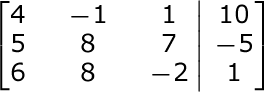Page 3

Question 11 11. This graph corresponds to which inequality?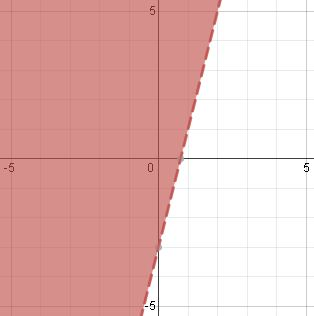Question 14 14. Which variable or variables need to be eliminated from the third equation in order to solve via the Gaussian elimination method?Question 15 15. What type of equation is this?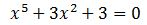Page 4

Question 18 18. What type of equation is this?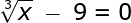Page 5

Question 25 25. What type of equation is this?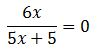Page 6

Question 28 28. Which variable in which equation needs to be eliminated in order to solve this system using Gaussian elimination?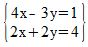CHSPE Mathematics: Patterns & Algebraic Equations Chapter Exam Instructions

Choose your answers to the questions and click 'Next' to see the next set of questions. You can skip questions if you would like and come back to them later with the yellow "Go To First Skipped Question" button. When you have completed the practice exam, a green submit button will appear. Click it to see your results. Good luck!

Support